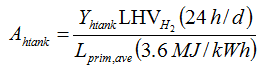# HOMER Pro 3.11

 Navigation: Glossary Hydrogen Tank AutonomyType: Output Variable Units: hr Symbol: Ahtank

The hydrogen tank autonomy is the ratio of the energy capacity of the hydrogen tank to the electric load. HOMER calculates the hydrogen tank autonomy using the following equation:where: Yhtank = capacity of the hydrogen tank [kg] LHVH2 = energy content (lower heating value) of hydrogen [120 MJ/kg] Lprim,ave = average primary load [kWh/d]